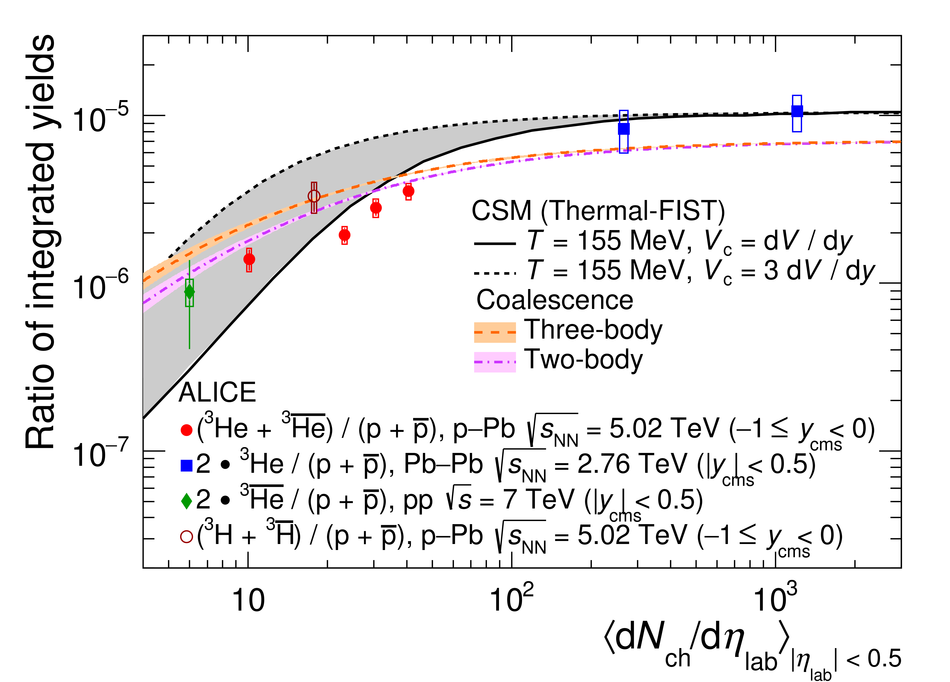# Figure 7

 $^3$He/p ratio in pp, p--Pb, and Pb--Pb collisions as a function of the mean charged-particle multiplicity density, together with the $^3$H/p ratio. Statistical and systematic uncertainties are indicated by vertical bars and boxes, respectively. The expectations for the canonical statistical hadronization model (Thermal-FIST ) and two coalescence approaches are shown. For the thermal model, two different values of the correlation volume are displayed The uncertainties of the coalescence calculations, which are due to the theoretical uncertainties on the emission source radius, are denoted as shaded bands.